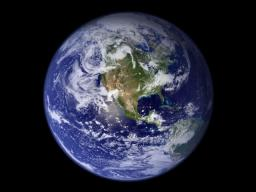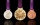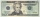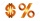# Earth's surface

The greater part of the earth's surface (r = 6371 km) is covered by oceans; their area is approximately 71% of the Earth's surface. What is the approximate area of the land?

Correct result:

x =  147918697 km2

#### Solution:We would be pleased if you find an error in the word problem, spelling mistakes, or inaccuracies and send it to us. Thank you!Tips to related online calculators

#### You need to know the following knowledge to solve this word math problem:

We encourage you to watch this tutorial video on this math problem:

## Next similar math problems:

• SummerjobThe temporary workers planted new trees. Of the total number of 500 seedlings, they managed to plant 426. How many percents did they meet the daily planting limit?
• RewardJanko and Peter divided the reward from the brigade so that Peter got 5/8 of the reward. What percentage of Janko's reward got?
• PersonsPersons surveyed:100 with result: Volleyball=15% Baseball=9% Sepak Takraw=8% Pingpong=8% Basketball=60% Find the average how many like Basketball and Volleyball. Please show your solution.
• Selling priceFind the selling price. Cost to store: \$50 Markup: 10%
• Real estate 2A real estate agent sold a lot for Php.550,000. If his agency pays at 10% commission, how much commission will he or she receive?
• The Chemistry testThe Chemistry test contained 8 questions, each with 3 points. Peter scored 21 points. How many percent did Peter write a test?
• WalkingOf the 450 students at the school, 432 walks to school. What percentage is this?
• Art schoolEvery fifth pupil 9A goes to art school. How many percent of pupils in class 9A go to art school?
• Percentages52 is what percent of 93?
• StudentsStudents solve word problems. They received 3 points for each correctly solved task. Four points were deducted for a task that was not solved or incorrectly solved. Tomas solved a total of 15 tasks and scored 24 points. How many tasks did he solve correct
• Apples 14Maya bought 18 apples. She used 50% of the apples to make apple pies and 1/3 of the apples was mixed with other fruits to make salad. How many apples were left?
• Profit gainIf 5% more is gained by selling an article for Rs. 350 than by selling it for Rs. 340, the cost of the article is:
• What percentWhat percent of 51 3/5 is 47 2/5?
• Fit ballWhat is the size of the surface of Gymball (FIT - ball) with a diameter of 65 cm?
• Price increase 2xIf two consecutive times we increase the price of the product by 20%, how many % is higher final price than the original?
• Cube surface areaThe surface of the cube was originally 216 centimeters square. The surface of the cube has shrunk from 216 to 54 centimeters sq. Calculate how much percent the edge of the cube has decreased.Alaska is the largest state in the United States and has a surface area of approximately 588,000 square miles. Montana has a surface area that is approximately 25% of the surface area of Alaska. What is the approximate surface area of Montana?